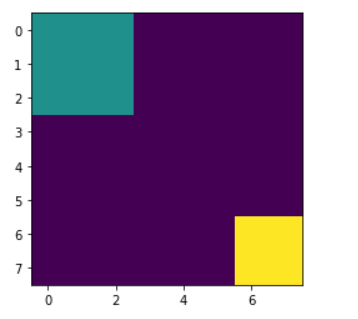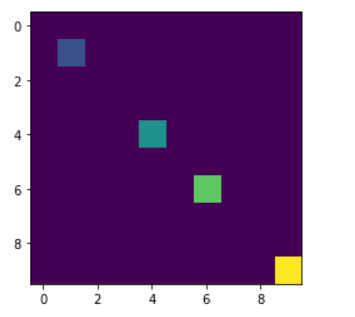# Labeled Image Function in Python Mahotas

In this article we will see how we can obtain a labeled function from a binary function in mahotas. Labeled images are integer images where the values correspond to different regions. I.e., region 1 is all of the pixels which have value 1, region two is the pixels with value 2, and so on. By convention, region 0 is the background and often handled differently.

In order to do this we will use `mahotas.label` method

Syntax : mahotas.label(regions)

Argument : It takes numpy.ndarray object as argument

Return : It returns numpy.ndarray object and integer value

Example 1:

 `# importing required libraries ` `import` `mahotas as mh ` `import` `numpy as np ` `from` `pylab ``import` `imshow, show ` ` `  `# creating region ` `# numpy.ndarray ` `regions ``=` `np.zeros((``8``, ``8``), ``bool``) ` ` `  `# setting 1 value to the region ` `regions[:``3``, :``3``] ``=` `1` `regions[``6``:, ``6``:] ``=` `1` ` `  `# getting labeled function ` `labeled, nr_objects ``=` `mh.label(regions) ` ` `  `# showing the image with interpolation = 'nearest' ` `imshow(labeled, interpolation ``=``'nearest'``) ` `show() `

Output :Example 2:

 `# importing required libraries ` `import` `mahotas as mh ` `import` `numpy as np ` `from` `pylab ``import` `imshow, show ` ` `  `# creating region ` `# numpy.ndarray ` `regions ``=` `np.zeros((``10``, ``10``), ``bool``) ` ` `  `# setting 1 value in the region ` `regions[``1``, ``1``] ``=` `1` `regions[``6``, ``6``] ``=` `1` `regions[``4``, ``4``] ``=` `1` `regions[``9``, ``9``] ``=` `1` ` `  `# getting labeled function ` `labeled, nr_objects ``=` `mh.label(regions) ` ` `  `# showing the image with interpolation = 'nearest' ` `imshow(labeled, interpolation ``=``'nearest'``) ` `show() `

Output :My Personal Notes arrow_drop_upCheck out this Author's contributed articles.

If you like GeeksforGeeks and would like to contribute, you can also write an article using contribute.geeksforgeeks.org or mail your article to contribute@geeksforgeeks.org. See your article appearing on the GeeksforGeeks main page and help other Geeks.

Please Improve this article if you find anything incorrect by clicking on the "Improve Article" button below.

Article Tags :

Be the First to upvote.

Please write to us at contribute@geeksforgeeks.org to report any issue with the above content.